# Noise Suppression Products/EMI Suppression Filters

Noise Suppression Basic Course Section 1
Chapter 1
Chapter 2
Chapter 3
Chapter 4
Chapter 5
Chapter 6
Chapter 6

## EMI suppression filters

#### 6-5-4. Skillfully using capacitors

##### (1) Adjust the self-resonant frequency with the frequency of the noise as a guide

The impedance of a capacitor is the minimum point of its self-resonant frequency. This may be a smaller value than that of the ideal capacitor at this frequency. If the frequency of the noise is fixed, it is possible to obtain a significant noise reduction effect, as long as the self-resonant frequency can be adjusted to match the noise.
In order to adjust the self-resonant frequency, select a capacitor with an electrostatic capacitance that matches the noise. As shown in Figure 4, if the electrostatic capacitance of the capacitor is changed, the characteristic curve where the capacitor is capacitive moves in parallel. As shown in Fig. 16, the self-resonant frequency also changes accordingly. The self-resonant frequency can thus be adjusted to match the frequency of the noise.
However, the self resonant frequency is affected and changed by the minute amount of inductance of the print board. This will be explained later. The frequency cannot be adjusted well on paper, so it is important to confirm the results during an actual test.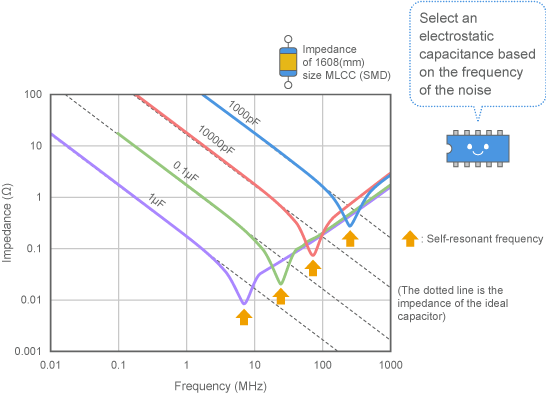##### (2) If the noise frequency is wide, the only choice is to use a capacitor with low ESL

When suppressing noise, it is generally difficult to estimate the noise frequency ahead of time. In this case, a filter that works on a wide range of frequencies must be prepared to ensure that the filter is effective no matter what the noise frequency is. Method (1) cannot be used for this kind of application.
Fig. 16 shows that the impedance in the area where the capacitor is inductive (at 1GHz, for example) hardly changes at all even if the electrostatic capacitance is changed. As explained earlier, this is because ESL accounts for much of the capacitor's impedance at these frequencies, and so it is difficult for electrostatic capacitance to exert an effect.
The only way to suppress noise across a wide range of high frequencies (at which the capacitor is inductive) is to use a capacitor with as little ESL as possible.

##### (3) ESL may have an effect even on low frequency noise

In Fig. 13, ESL had an effect only at frequencies above 100MHz. However, there may be cases where ESL exerts a strong effect even at extremely low frequencies, when using a capacitor with a large electrostatic capacitance. This is because the self-resonant frequency is reduced, so the frequency range at which the capacitor is inductive spreads to the lower frequencies.
Fig. 17 shows examples where the ESL was changed on a capacitor with an electrostatic capacitance of 10μF. This shows that ESL can exert a strong effect even at what are considered low frequencies when it comes to noise suppression (such as 1MHz) . In cases like this where the expected noise reduction effect cannot be obtained with a large capacity capacitor, there is room to consider whether or not ESL is exerting an effect.
Note however that the ESL of a bypass capacitor includes not only the ESL of the capacitor itself, but the inductance of the wiring used to install the parts. This will be explained in the next section. Therefore, when attempting to reduce the ESL, it is also important to consider the wiring used to install the capacitor.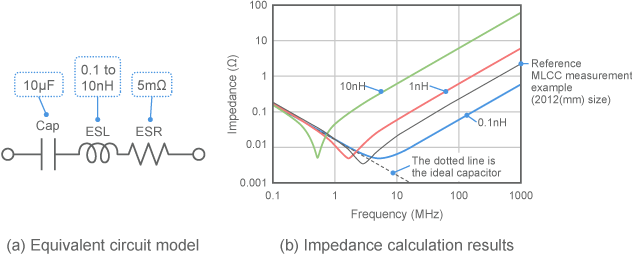Fig. 17 Effect of ESL on large capacity capacitors

##### (4) The pattern used to install the capacitor can be a factor behind ESL

It was mentioned earlier that ESL has a significant effect on a capacitor's noise reduction effect. However, there is one other factor that plays a large role. This is the inductance generated when installing the capacitor on a board.
As shown in Fig. 18, a wiring pattern and via are required to install the capacitor to the board and connect it to the circuit. The inductance of these parts is added in series to the bypass capacitor. Therefore, when the capacitor is installed on a board and actually operated, the overall ESL of the bypass circuit will be greater than the ESL of the capacitor itself.
This "overall ESL" is what has an effect on the actual noise reduction result.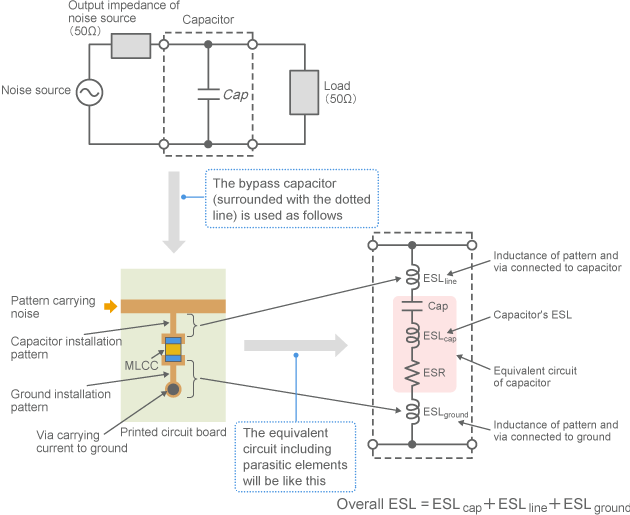Fig. 18 Overall ESL of bypass circuits

##### (5) What is the effect of the pattern and via inductance?

What is the impact of the wiring and via inductance? It is actually large enough that it cannot be ignored—in fact, the effect might even be greater than that of the ESL of the capacitor itself, depending on how it is being used.
Fig. 19 shows the results of calculating the impedance when a 1mm wide pattern is installed on both ends of the capacitor. This shows that even wiring that is only several mm wide can increase the impedance at 100MHz ten times over or more.
When using a capacitor to reduce high frequency noise, the pattern must be kept short.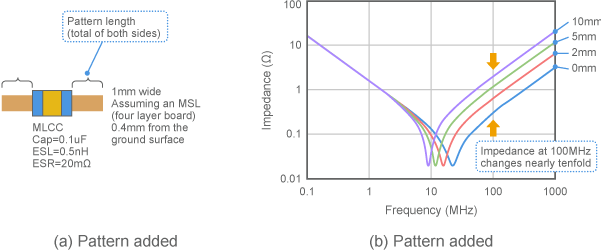Fig. 19 Capacitor impedance including pattern (calculated values)

##### (6) Approximate pattern and via inductance

How much ESL do patterns and via carry? The amount is extremely small and cannot easily be measured or calculated. However, a pattern will generally have around 0.5nH per 1mm, while a via will generally have 0.1nH per unit. (Either case assumes comparatively good conditions with a multi-layer board and a ground plane found 0.4mm below. The values will increase if using a double-sided board or the like.)
Considering the fact that the ESL of the capacitor itself will be around 0.5nH (even if an MLCC is used) , these values are large enough that they cannot be ignored. The capacitor must be installed in such a way that minimizes this inductance, if the capacitor is to be used effectively.

##### (7) How to install capacitors to minimize ESL

Fig. 20 (b) and (c) show examples of installations where pattern and via inductance are reduced.
Connect a ground plane or other solid ground to the capacitor. In this figure, a board with a built-in ground plane is used.
Place the via that connects the capacitor and ground plane close to the capacitor. If possible, arrange several vias around the capacitor, as shown in the figure. Placing the via below the capacitor will further reduce ESL.
Fig. 20 (b) shows an example where the capacitor is placed directly over the wiring carrying the noise, with the wiring kept as short as possible. Doing so will reduce the pattern and via inductance to a level that can be ignored.
However, this method requires you to move the location of the via connected to the ground.
Fig. 20 (c) shows an example of a solution for when the location of the via cannot be moved. As shown in the figure, the capacitor is placed close to the via connected to ground, while the pattern carrying the noise is moved close to the capacitor. Doing this can minimize the inductance in the direction noise is bypassed.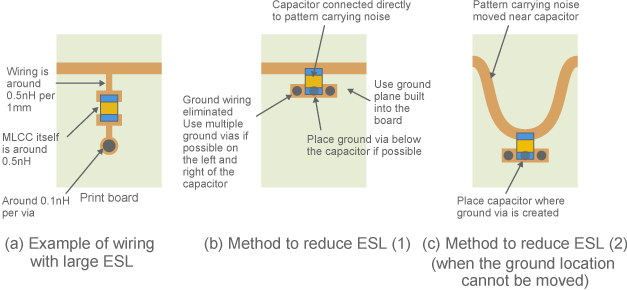Fig. 20 Using capacitors that reduce ESL

##### (8) Use a dedicated capacitor to eliminate noise exceeding 1GHz

The measurement results in Fig. 17 show that ESL must be around 0.2nH or less in order to obtain an impedance of 1 ohm or less at 1GHz. This value is so small that it would be impossible even with an MLCC. You must also consider the inductance of the wiring and via, as shown in Fig. 18. So how should it be installed?
A three-terminal capacitor ( (5) in Fig. 12) can be used to solve this kind of problem. Capacitors with low ESL (such as three-terminal capacitors) are used to suppress noise in frequencies at 1GHz and higher. More details will be provided in Chapter 8.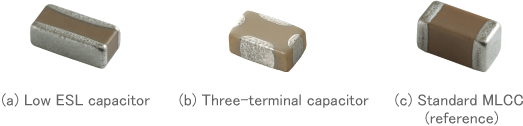Fig. 21 Capacitors with low ESL

Top of page

### Actual characteristics of capacitors" - Key points

• Actual capacitors contain two types of parasitic elements: ESL and ESR.
• The effect of parasitic elements is larger than the electrostatic capacitance when eliminating noise at high frequencies.
• Use capacitors with low ESL to eliminate a wide range of noise above 100MHz.
• Note that ESL is also generated from the pattern used to install the capacitor.